#### Option 3.2.7. Trans-High Accum

Select Trans_High Accum in the Calculation Type field on the Virtual tab.

A Trans-High Accum point accumulates the number of times the Expression value transitions from a zero to a non-zero value. While the CIMPLICITY project is running, the Trans_High Accum point remembers its latest state, even if the points it depends on become unavailable.

Determining a transition takes into consideration the calculation type of the expression and the point type of the Trans-High Accum point.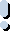Important: Transition points are not supported in redundancy.

Example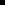If the Trans-High Accum point type is DINT and the Expression uses floating point arithmetic, the result of the calculation is rounded to the nearest integer. For example, a value of 0.1 is considered to be zero, and a value of 0.6 is considered to be non-zero.If the Trans-High Accum point type is FLOAT and the Expression uses floating point arithmetic, then a transition from 0 to 0.1 is considered to be a transition from a zero to a non-zero value.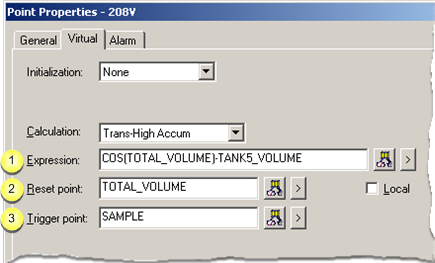Expression Reset point Trigger point

 Expression

Enter an expression in the Expression field on the Virtual tab of the Point Properties dialog box.

Enter an expression that calculates the value of the Trans-High Accum point.

The expression will be monitored for transitions.

The expression may contain:One or more Point IDs as well asConstant values, operations, and functions.

Buttons to help with the equation are as follows.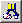Opens the Expression Editor. Displays a Popup menu to: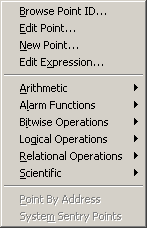Open: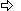The Select a Point browser.The Point Properties dialog box for a selected point.A New Point dialog box.The Expression Editor.Display an extended menu with the associated operations.

 Reset point

Based on whether there is an entry in the Reset Point field, at runtime the Trans-High Accum point is reset as follows:

 When the trigger point is not defined If And Then An Initial Trans High Accum Value is The Current value of the Expression is The Trans High Accum point value is reset to the: Not defined Unavailable Zero Not defined Available Zero Defined Unavailable Initial value Defined Available Initial value When the trigger point is defined If Then An Initial Trans High Accum Value is The Trans High Accum point value is reset to the: Not defined Zero Defined Initial value

Note: Buttons to the right of the Reset Point and Trigger Point fields help with configuration.Opens the Select a Point browser to select an available point. Displays a Popup menu to: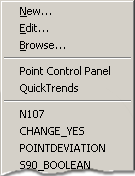Open:A New Point dialog box.The Point Properties dialog box for a selected point.The Select a Point browser.Select a recently selected point.

 Trigger point

When the value of the Trans-High Accum point updates depends on whether or not you defined the trigger point as follows:

 Trigger point The Value of the Min Capture Point is Updated When the Value of the: Defined Trigger point is updated. Not defined Source point in the Expression field is updated.

Buttons to help with the trigger point have the same functionality as the buttons to the right of the Reset Point field.

 Step 3.2. Configure virtual calculations.